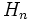# P-simple IAPS

BEWARE! This term is nonstandard and is being used locally within the wiki. [SHOW MORE]
This term is related to: APS theory
View other terms related to APS theory | View facts related to APS theory
ANALOGY: This is an analogue in IAPS of a property encountered in group. Specifically, it is a IAPS property analogous to the group property: simple group
View other analogues of simple group | View other analogues in IAPSs of group properties (OR, View as a tabulated list)

This article defines a property that can be evaluated for an IAPS of groups

## Definition

### Symbol-free definition

An IAPS of groups is termed p-simple if it has no strongly proper nontrivial normal sub-IAPS.

### Definition with symbols

An IAPS of groups$(G,\Phi)$ is termed p-simple if there is no sub-IAPS$H$ of$G$ satisfying all these conditions:

•$H_n \triangleleft G_n$ for every$n$
• There are infinitely many indices$n$ for which$H_n$ is properly contained in$G_n$
•$H_n$ is nontrivial for at least some value of$n$